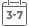# Matrix Analysis for Statistics

• Publish Date: 2005-01-12
• Binding: Hardcover
• Author: James R. Schott
• Sale
• \$52.87
• Regular price \$109.38A complete, self-contained introduction to matrix analysis theory and practice

Matrix methods have evolved from a tool for expressing statistical problems to an indispensable part of the development, understanding, and use of various types of complex statistical analyses. This evolution has made matrix methods a vital part of statistical education. Traditionally, matrix methods are taught in courses on everything from regression analysis to stochastic processes, thus creating a fractured view of the topic. This updated second edition of Matrix Analysis for Statistics offers readers a unique, unified view of matrix analysis theory and methods.

Matrix Analysis for Statistics, Second Edition provides in-depth, step-by-step coverage of the most common matrix methods now used in statistical applications, including eigenvalues and eigenvectors; the Moore-Penrose inverse; matrix differentiation; the distribution of quadratic forms; and more. The subject matter is presented in a theorem/proof format, allowing for a smooth transition from one topic to another. Proofs are easy to follow, and the author carefully justifies every step. Accessible even for readers with a cursory background in statistics, yet rigorous enough for students in statistics, this new edition is the ideal introduction to matrix analysis theory and practice.

The book features:

• Self-contained chapters, which allow readers to select individual topics or use the reference sequentially
• Extensive examples and chapter-end practice exercises, many of which involve the use of matrix methods in statistical analyses
• New material on elliptical distributions and new expanded coverage of such topics as eigenvalue inequalities and matrices partitioned in 2 by 2 form, in particular, results relating the rank, generalized inverse, eigenvalues of such matrices to their submatrices, and much more